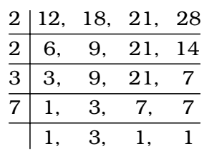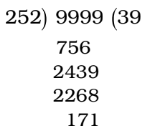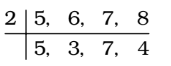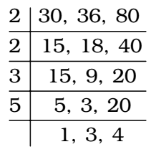## LCM and HCF

#### LCM and HCF

1. A number p is divisible by 7. When this number is divided by 8, 12 and 16. It leaves a remainder 3 in each case. The least value of p is:

1. We get LCM of 8, 12 and 16 = 48
∴ Required number = 48a + 3 which is divisible by 7.
∴ p = 48a + 3 = (7 × 6a) + (6a + 3) which is
divisible by 7.
i.e. 6a + 3 is divisible by 7.
When a = 3, 6a + 3 = 18 + 3 = 21 , which is divisible by 7.

##### Correct Option: D

We get LCM of 8, 12 and 16 = 48
∴ Required number = 48a + 3 which is divisible by 7.
∴ p = 48a + 3 = (7 × 6a) + (6a + 3) which is
divisible by 7.
i.e. 6a + 3 is divisible by 7.
When a = 3, 6a + 3 = 18 + 3 = 21 , which is divisible by 7.
∴ p = 48 × 3 + 3 = 144 + 3 = 147
Hence , The least value of p is 147 .

1. The greatest four digit number which is exactly divisible by each one of the numbers 12, 18, 21 and 28 is

1. First of all , we find the LCM of 12 , 18 , 21 and 28∴ LCM = 2 × 2 × 3 × 3 × 7 = 252
The largest 4-digit number = 9999Here , Remainder = 171
∴ Required number = The largest 4-digit number - Remainder

##### Correct Option: A

First of all , we find the LCM of 12 , 18 , 21 and 28∴ LCM = 2 × 2 × 3 × 3 × 7 = 252
The largest 4-digit number = 9999Here , Remainder = 171
∴ Required number = The largest 4-digit number - Remainder
Hence , Required number = 9999 – 171 = 9828

1. Let p be the least number, which when divided by 5, 6, 7 and 8 leaves a remainder 3 in each case but when divided by 9 leaves no remainder. The sum of digits of p is

1. We find LCM of 5, 6, 7 and 8 = 840∴ LCM = 2 × 5 × 3 × 7 × 4 = 840
∴ Required number = 840p + 3 which is divisible by 9 for a certain least value of p.
Now, 840p + 3 = 93p × 9 + 3p + 3
∴ 3p + 3 , is divisible by 9 for p = 2

##### Correct Option: C

We find LCM of 5, 6, 7 and 8 = 840∴ LCM = 2 × 5 × 3 × 7 × 4 = 840
∴ Required number = 840p + 3 which is divisible by 9 for a certain least value of p.
Now, 840p + 3 = 93p × 9 + 3p + 3
3p + 3 , is divisible by 9 for p = 2
∴ Required number = 840 × 2 + 3 = 1680 + 3 = 1683
∴ Sum of digits = 1 + 6 + 8 + 3 = 18

1. The number between 3000 and 4000 which is exactly divisible by 30, 36 and 80 is

1. Firstly, we find the LCM of 30, 36 and 80.∴ LCM = 2 × 2 × 3 × 5 × 3 × 4 = 720

##### Correct Option: D

Firstly, we find the LCM of 30, 36 and 80.∴ LCM = 2 × 2 × 3 × 5 × 3 × 4 = 720
∴ Required number = Multiple of 720 = 720 × 5 = 3600 ; because 3000 < 3600 < 4000

1. HCF and LCM of two numbers are 7 and 140 respectively. If the numbers are between 20 and 45, the sum of the numbers is :

1. Let the numbers be 7p and 7q where p and q are co-prime.
Now, LCM of 7p and 7q = 7pq
∴ 7pq = 140

 ⇒ pq = 140 = 20 7

Now, required values of p and q whose product is 20 and are co-prime, will be 4 and 5.

##### Correct Option: C

Let the numbers be 7p and 7q where p and q are co-prime.
Now, LCM of 7p and 7q = 7pq
∴ 7pq = 140

 ⇒ pq = 140 = 20 7

Now, required values of p and q whose product is 20 and are co-prime, will be 4 and 5.
∴ Numbers are 28 and 35 which lie between 20 and 45.
∴ Required sum = 28 + 35 = 63.